## Monday, February 12, 2018

### Automatically resizing the tip labels of a plotted tree

It just occurred to me that it might be useful for some users if the font size for our tree by default scaled automatically with the number of tips in our tree and the dimensions of our open plotting device.

Here's a code chunk that will do this. It's very simple:

``````foo<-function(tree,...){
fsize<-36*par()\$pin/par()\$pin/Ntip(tree)
plotTree(tree,fsize=fsize,lwd=1,...)
}
``````

I chose 36 as the number of taxa to measure against, because 36 results in a nice tip label spacing for `cex=1.0` with the stardard plotting device dimensions. E.g.:

``````library(phytools)
tree<-pbtree(n=36)
plotTree(tree)
``````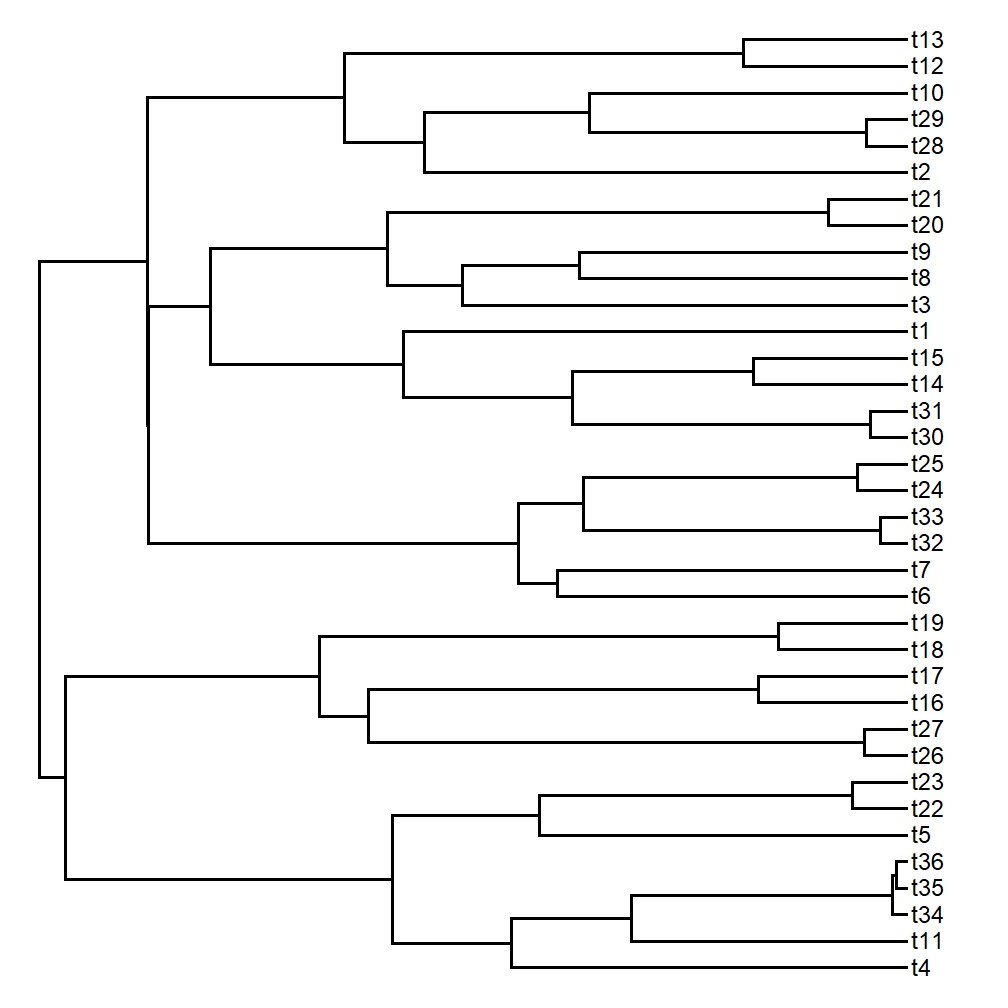Let's try some different tree sizes:

``````foo(pbtree(n=20))
``````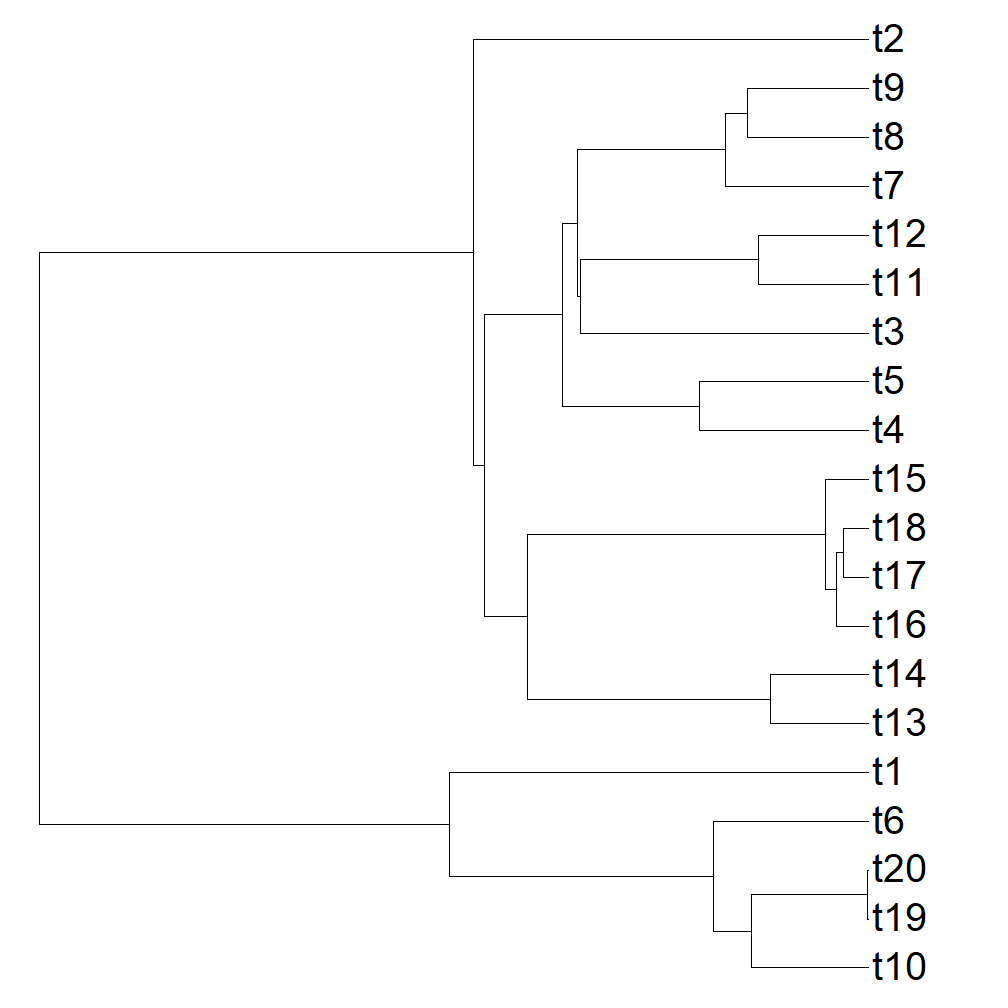``````foo(pbtree(n=80))
``````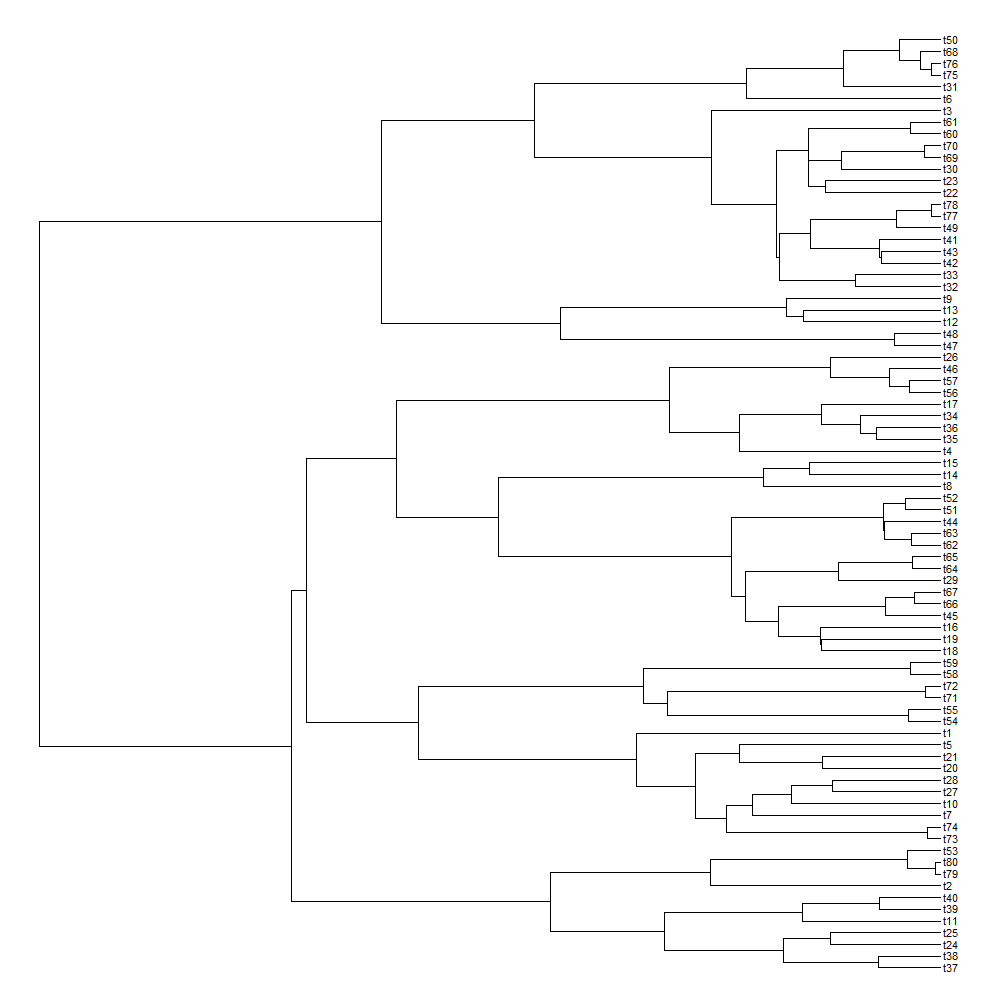``````data(anoletree)
foo(anoletree,ftype="i")
``````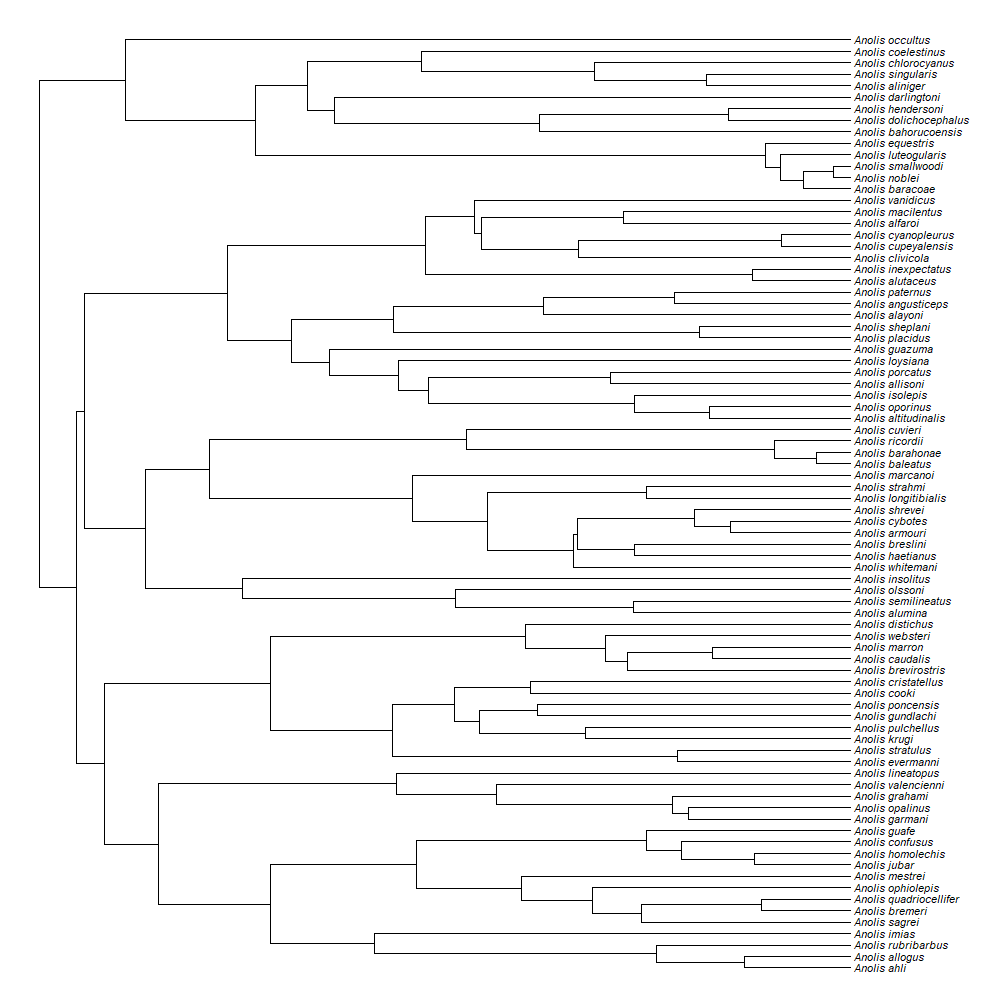The piece `par()\$pin/par()\$pin` computes the aspect ratio of the current plotting device so that the font can be resized as appropriate. For example (for a 6 W by 10.5 H plot area):

``````foo(anoletree,ftype="i")
``````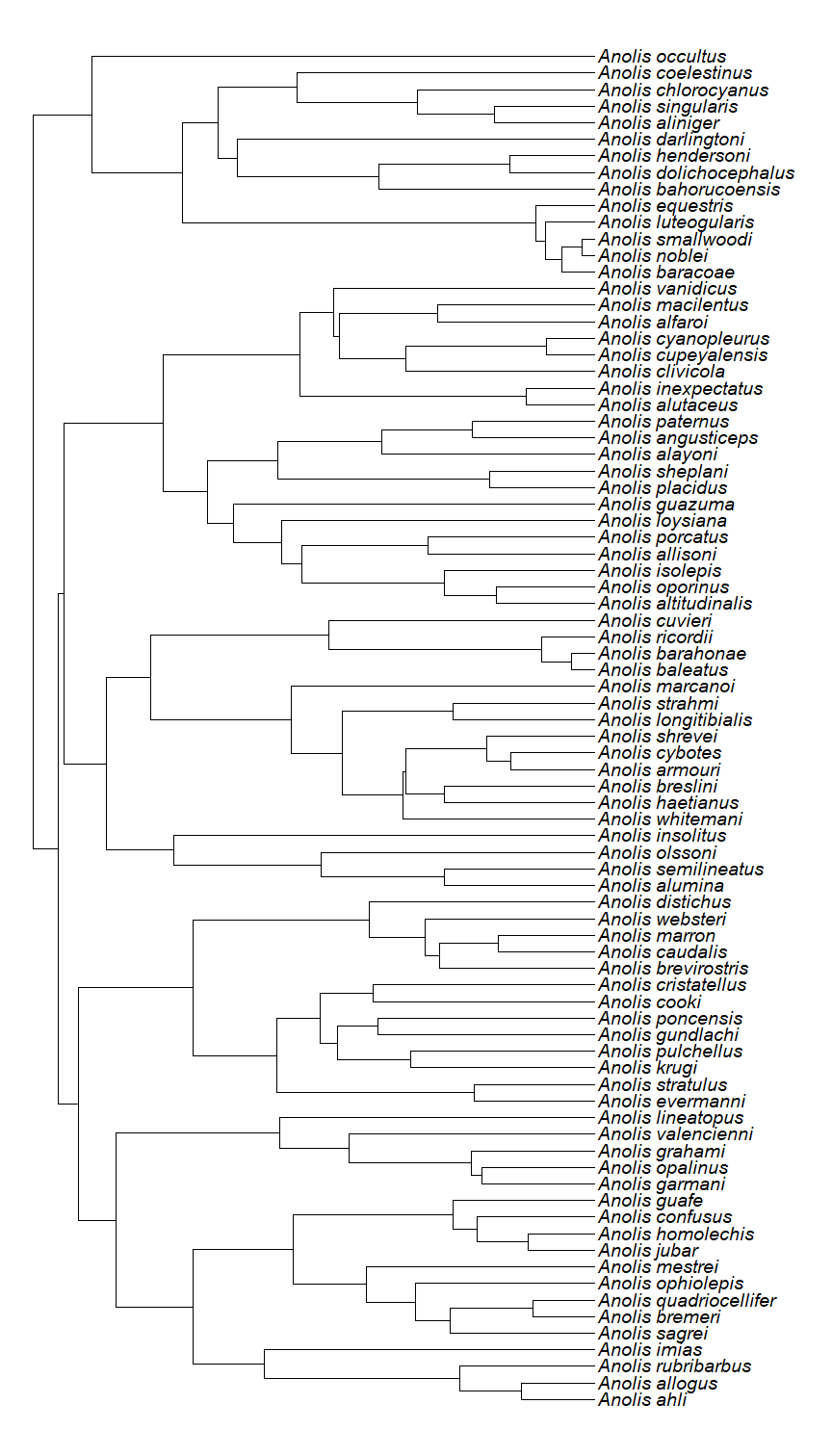or (for a plot area shorter than it is tall):

``````foo(pbtree(n=40))
``````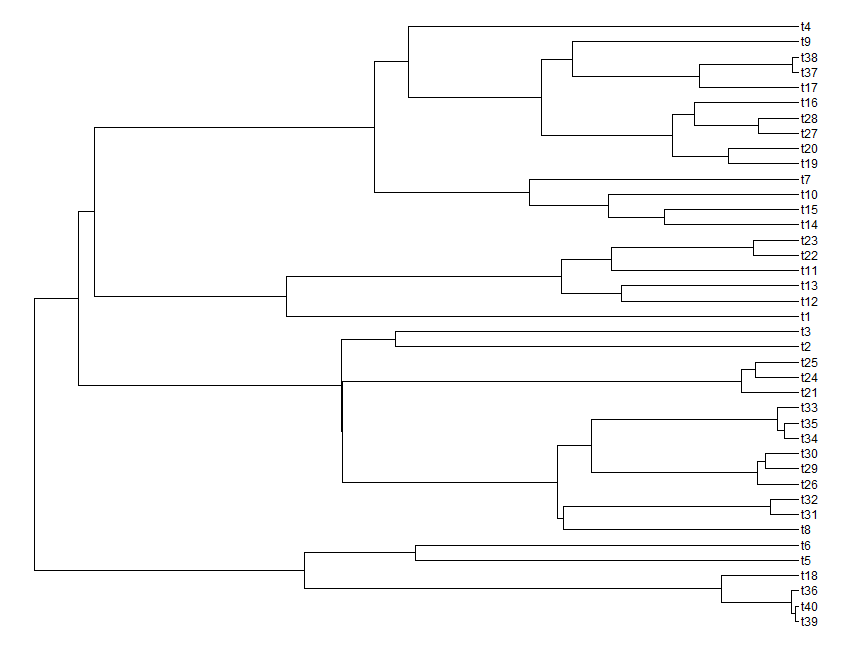It is imperfect, in large part because (depending on our plotting device) the plotted font size does not actually change continuously in R.

I'm going to use this in another new phytools plotting function shortly.

That's it.

1.Good afternoon,

I was wondering if this is currently a built in function within the phytool package.

-Teisha King

2.I am interested in the format of your "data(anoletree)". Is this a .csv or tab-delimited file? I am trying to adapt my own data to the Bayesian stochastic mapping script and I get hung-up at this point.

Note: due to the very large amount of spam, all comments are now automatically submitted for moderation.